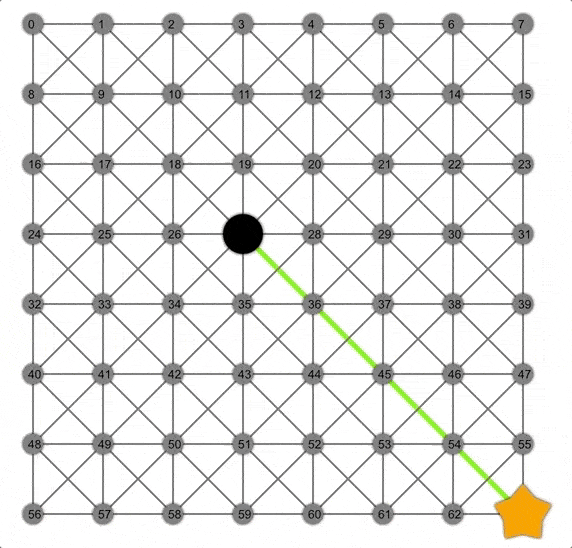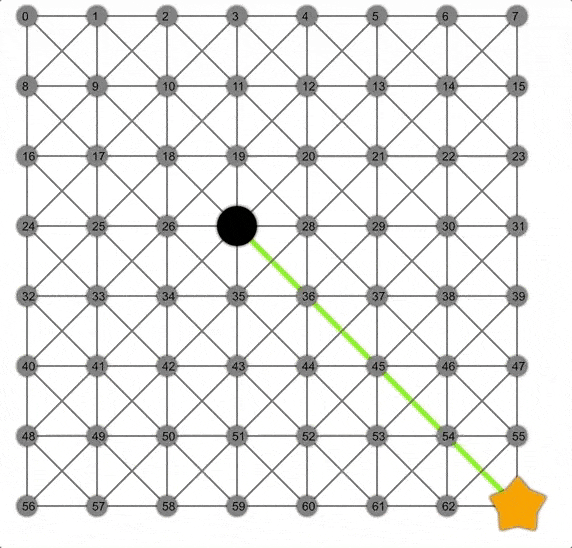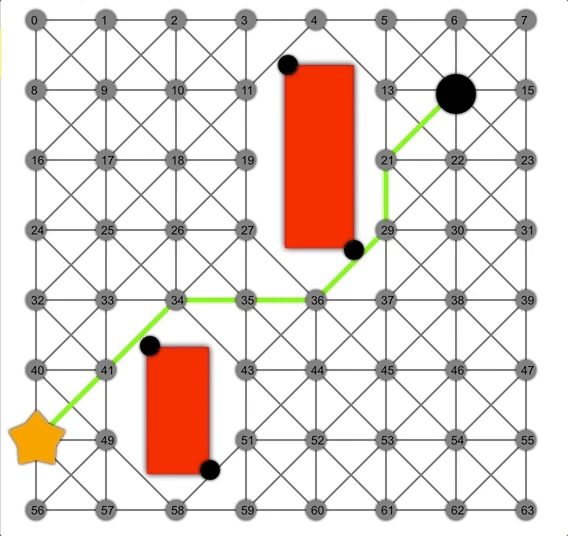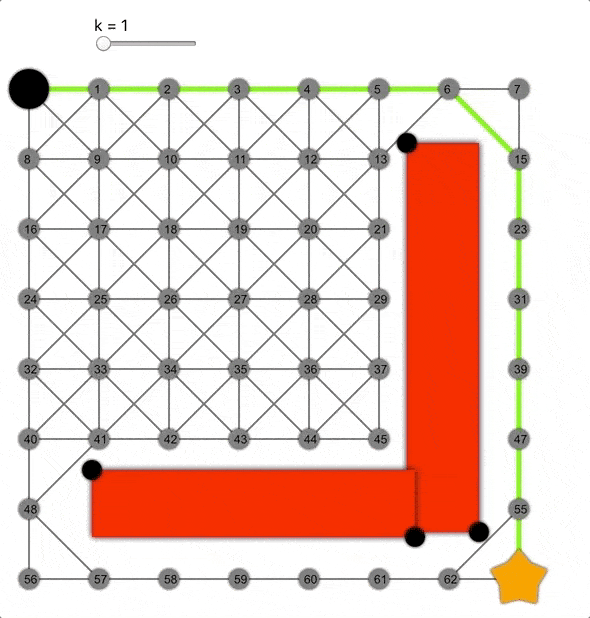# Interactive pathfinding

Link to hosted demo: https://interactive-pathfinding.netlify.com/

Breadth-first search, Dijkstra and A* are three famous path-planning algorithms that run on graphs. They can all be seen as a specialised version of a graph search with two different parameters, the queue used and the heuristic used.

The aim of this project is to explore and visualise how the different algorithms explore through the graph depending of the parameters chosen.

The general algorithm works as follow:

A frontier is initialised as a queue containing the start node.

While the frontier is not empty a node (called "current") is removed from the queue and gets "visited". Each of the neighbours of the visited node gets added to the frontier with a cost which is the current cost of getting to the current node plus the cost of visiting the neighbour from the current node plus the value of an heuristic function applied to the neighbour and the goal node. The heuristic function is an estimation of the cost of the path from the two nodes.

A reference of the direction of the visit is stored (usually in a cameFrom map) in order to be able to reconstruct the path when the algorithm stops. If the neighbour is already in the frontier its cost can be changed if the new path has a cheaper cost.

The algorithm stops when it finds a path to the goal (early exit) or when the frontier is empty.

## BFS

BFS can be implemented by using a First-In-First-Out queue. This kind of queue ignores the cost of the links in the path and it expands based on the number of hops. Because of this it's guaranteed to find the shortest path in terms of hops, but not in terms of costs associated with the hops. The heuristic used can be whatever we want, as it will be ignored by the queue.

The fifo has been implemented using an array, appending elements to the end and removing them from the start.Animation of BFS, notice how in a grid the frontier (yellow nodes) expands as a square, because a square is the set of the nodes at the same "hop-distance"

## Dijkstra

By passing a PriorityQueue instead of a FIFO to the graph and a heuristic function which always returns 0 we get the Dijkstra algorithm.

The main difference from the BFS is that Dijkstra takes into account the costs, the algorithm can now find the actual shortest path considering costs of traveling from node to node.

The priority queue has been implemented with an array that get sorted after every insertion. While not being the most efficient implementation of a priority queue, it was easier to implement and is fast enough for this application.Animation of Dijkstra, notice how the frontier is now a circle.

## A*

To obtain the A* algorithm from our generalised graph search we just need to pass an actual heuristic function, let's use the euclidean distance between the two nodes as example. By weighting the nodes based on "cost to the node" + "estimation of cost from node to goal" we can speed up the search by going first into nodes that look promising.Thanks to the heuristic, A* can find the correct path faster than Dijkstra or BFS

## Non admissible heuristics

A* is guaranteed to find the shortest path only if the heuristic is admissible, which means that it never overestimates the actual path length. The euclidean distance cannot overestimate, as the euclidean distance is the shortest distance/path between two points.

But what if we multiply it by a constant k > 0 ? By doing so it would overestimate making the heuristic non-admissible.The more we increase the value of k the more the algorithm goes towards the goal. This also makes it less accurate, making the resulting path not always the shortest.

## Implementation

The project was implemented in javascript in order to be more accessible on the web. I used react for rendering the UI and react-konva for rendering the graph.

The pathfinder is implemented as a function that accepts the queue type, the heuristic, and returns another function which is the actual pathfinder (this concept is known as currying).

In this way every time the user changes the settings, a new pathfinder function is created with the correct parameters and can be used to navigate the graph.

In order to show every step of the exploration, the function is a javascript generator, meaning that it returns an iterator and not just a single value. This allows me to yield the entire state of the algorithm at every step, save it into an array and then show a specific state based on the value of the slider at the top of the page.

Get A Weekly Email With Trending Projects For These Topics
No Spam. Unsubscribe easily at any time.
javascript (70,419
pathfinding (42
dijkstra (18